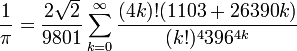Ramanujan's Formula for π

He was such an oddity. All of the earlier formulas for calculating π involved mostly simple numbers. But look at Ramanujan's:$\frac{1}{\pi} = \frac{2\sqrt{2}}{9801} \sum^\infty_{k=0} \frac{(4k)!(1103+26390k)}{(k!)^4 396^{4k}}$

It makes sense that someone who instantly could see what a special number 1729 is could come up with a formula like the above.

1.I think he has several of those, and I think I've read somewhere that they all seem to be right, but in some cases no one has been able to actually prove they are right.

Now that's a real genius.